# Slope of a Linear Function

HIDE SOLUTIONS

1. Find the slope of the following line:

y = 3x

2. Find the slope of the following line:

5y – 3x  = 4

Solution

Rewrite in the form y  =  a  +  bx3. Find the slope of the line segment connecting the following points:

(3,-1) and (-2,5)

x1  =  3              y1  =  1

x2  =  - 2            y2  =  5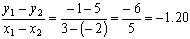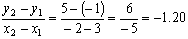4. Find the slope of the line segment connecting the following points:

(-1,-1) and (1,3)

x1  =  -1             y1  =  -1

x2  =   1             y2  =  3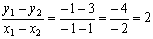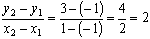5. Find the slope of the line segment connecting the following points:

(-4,2) and (1,2)

Answer:  slope is 0.  The line is horizontal.

x1  =  -4             y1  =  2

x2  =   1             y2  =  2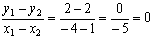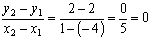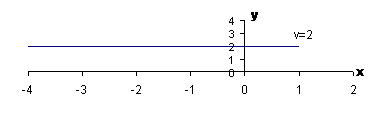6. Find the slope of the line segment connecting the following points:

(-3,3) and (-3,-2)

Answer:  slope is infinite.  The line is vertical.

x1  =  -3             y1  =  3

x2  =   -3            y2  =  -2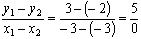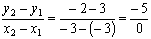[Index]